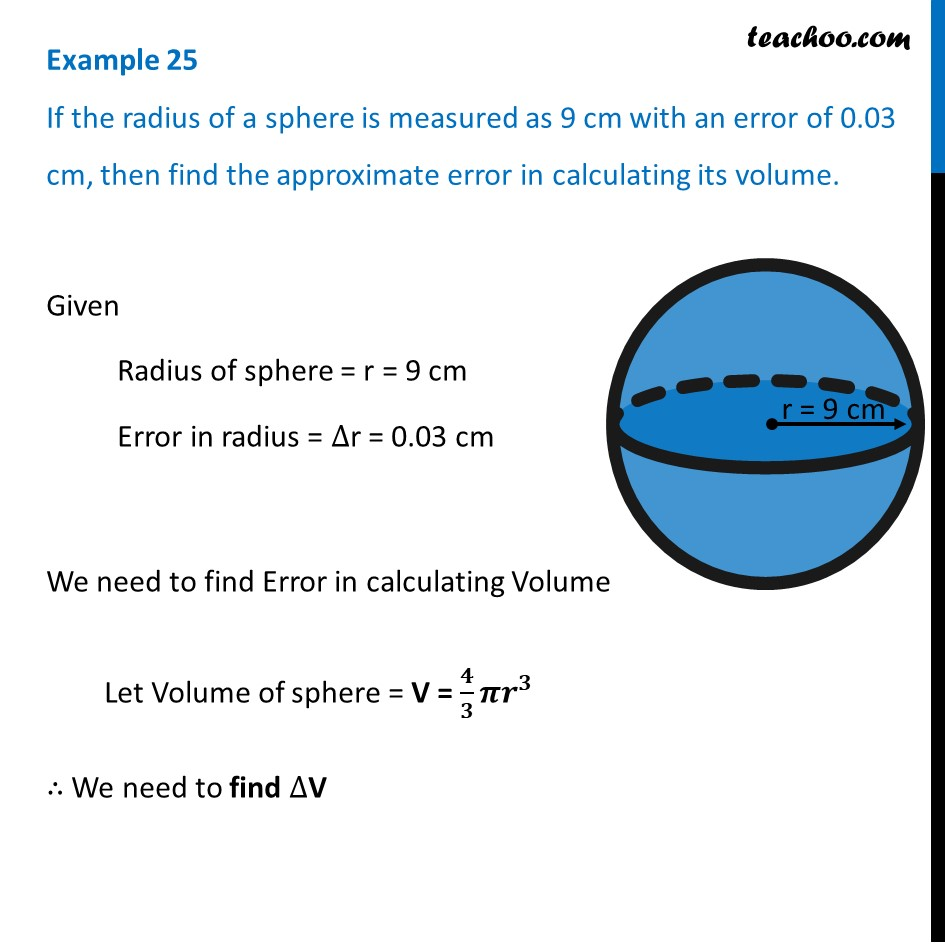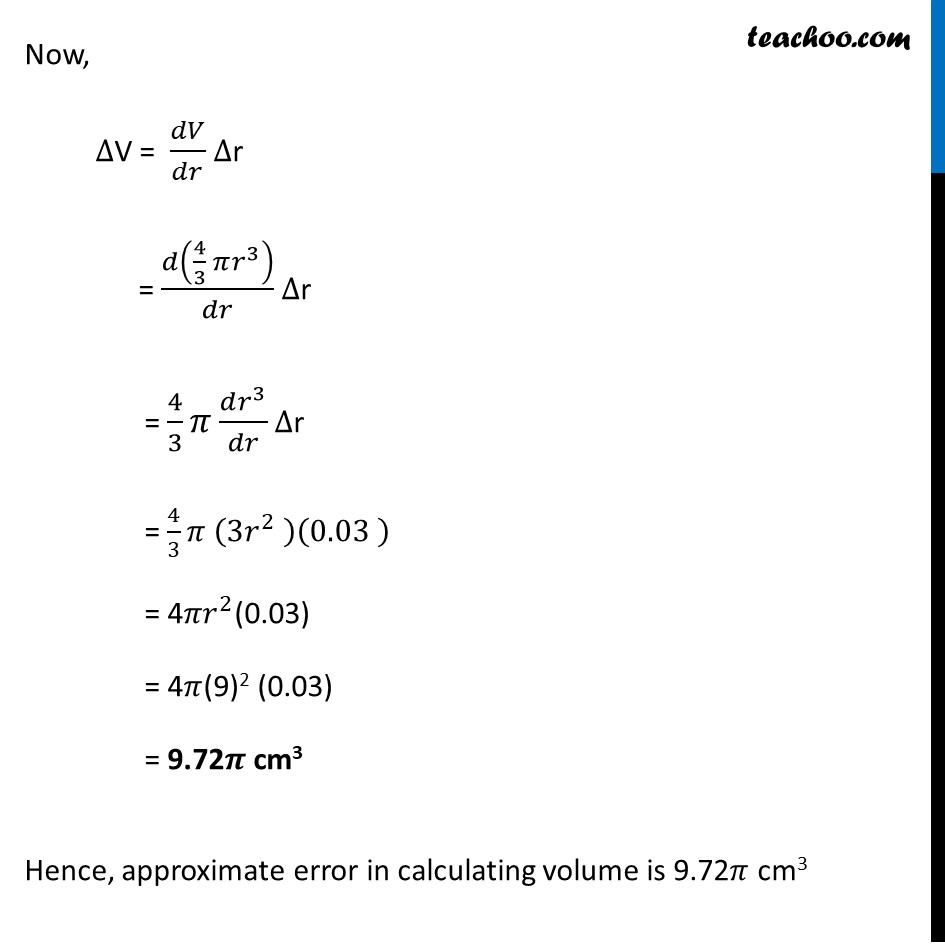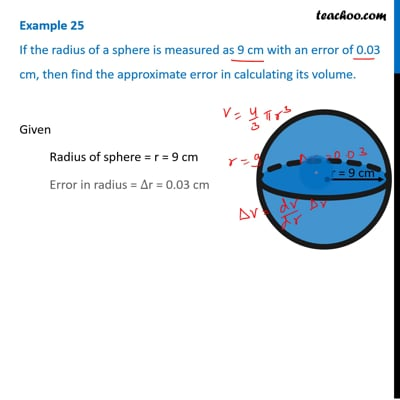Examples

Chapter 6 Class 12 Application of Derivatives
Serial order wiseThis video is only available for Teachoo black users

Solve all your doubts with Teachoo Black (new monthly pack available now!)

### Transcript

Example 25 If the radius of a sphere is measured as 9 cm with an error of 0.03 cm, then find the approximate error in calculating its volume.Given Radius of sphere = r = 9 cm Error in radius = ∆r = 0.03 cm We need to find Error in calculating Volume Let Volume of sphere = V = 𝟒/𝟑 𝝅𝒓^𝟑 ∴ We need to find ∆V Now, ∆V = 𝑑𝑉/𝑑𝑟 ∆r = 𝑑(4/3 𝜋𝑟^3 )/𝑑𝑟 ∆r = 4/3 𝜋 (𝑑𝑟^3)/𝑑𝑟 ∆r = 4/3 𝜋 (3𝑟^2 )(0.03 ) = 4𝜋𝑟^2(0.03) = 4𝜋(9)2 (0.03) = 9.72𝝅 cm3 Hence, approximate error in calculating volume is 9.72𝜋 cm3